# Solution assignment 09 Logarithmic equations

### Assignment 9

Solve: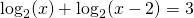### Solution

The domain is: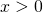and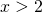thus:We write the equation as follows: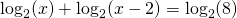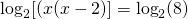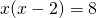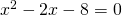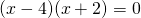The solutions of this equation are: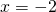or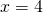The second solution lies in the domain and is valid. The first solution lies outside the domain and does not satisfy the equation.